# Here’s why the fundamental constants are not fundamental

Planck’s constant and the gravitational constant are considered fundamental constants of nature. Both are instrumental in calculating physical quantities, but what makes them fundamental? Are they truly irreducible quantities from first principles, or just misunderstood?

A close examination of the constants shows why they are not fundamental, but made from fundamental units.

### Reason #1: The traditional constants embody natural Planck units

Max Planck had it backwards when he defined natural units of length, mass, and time from ℏ, 𝐺 and 𝑐. Turning this assumption on its head gives us simple definitions for the two constants, evident from their unit dimensions and values.

### Gravitational constant

The traditional constants have always had complex unit dimensions because they convert formula inputs into the correct dimensions of a formula’s output. But the composition of natural units—the reason these transformations work—has remained a mystery.

### Reason #2: Planck units reveal meaningful structure

Planck’s constant and the gravitational constant could be characterized as magic numbers because they produce the right answers without revealing their methods. Restating these constants in natural units brings their secret to light.

Natural formulas have two parts. One part is the maximum potential of the unit dimensions we are solving for—quantified in Planck units. The second part includes one or more dimensionless, proportionality operators that reduce the unit potential in the proper ratio. For example, the equation for gravitational acceleration

accelerationrestated in natural units exposes the formula’s elementary structure:

Historical equations give the right answers because hidden ratios of Planck units create the required operators and potentials. It is the Planck units and not the composite values of the traditional constants that serve as constants of proportionality. This pattern repeats itself in one formula after another and gives physical meaning to ambiguous equations.

The following natural formulas illustrate the operators and potentials hidden by the composite structure of ℏ and 𝐺. Each formula is produced by replacing the traditional constants with equivalent natural units.

### natural formula

Compton wavelength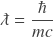de Broglie wavelength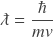momentum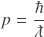photon energygravitational acceleration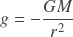gravitational forcegravitational energy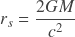orbital velocityescape velocity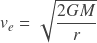Equations for gravitational force and energy expose a hidden quantity of Planck mass in the formulas’ numerators and denominators.

### Reason #3: Formulas written in Planck units are more efficient

What does efficiency have to do with being fundamental? Try creating certain ratios of Planck units using only composite constants. The formula for black hole temperature shows the kind of gymnastics required to produce 𝑚𝑃 𝐸𝑃 out of ℏ, 𝐺 and 𝑐. The equation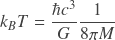has an enigmatic ratio between ℏ, 𝐺 and 𝑐3. The meaning of these constants is unclear until we restate the equation in elementary formproducing the natural formula

Restating the equation in elementary form shows the complicated ratios of traditional constants needed to produce the right mix of Planck units. The traditional formula introduces superfluous units simply to offset unnecessary quantities embedded in the constants.

Similarly, Planck’s derived equations for length, mass, and time require excessive ratios of the composite constants to isolate a single, fundamental unit. Here’s the formula for Planck length in elementary form:Formulas written in natural units do not contain unnecessary terms. They can be written in simplest form without excess baggage.

Combinations of the traditional constants are sometimes viewed as a convergence of quantum and classical systems. In fact, there is nothing inherently classical about the gravitational constant, or quantum mechanical about Planck’s constant. The real constants of proportionality—the Planck units—give a smooth transition between systems of any size in the simple ratios of a system’s properties to the Planck scale.

### Reason #4: Natural Planck units give meaningful explanations

Mathematics are suitable for natural phenomena when the symbols and operations represent real objects and relationships. But the physical meaning of formulas using ℏ and 𝐺 is unclear. Natural units of length, mass, and time, on the other hand, reveal relationships between mathematical symbols and the physical dynamics they describe.

Take the following expression of kinetic energy easily derived from natural formulas for wavelength, momentum, and energy.

### Electron

The formula shows a consistent structure underlying massive and massless particles, and a simple 2-part mechanism regulating the kinetic energies of both particle types. The mechanism elegantly characterizes kinetic energy as the product of wavelength potential and velocity.

Natural formulas also reconcile classical and quantum mechanical equations that appear different on the surface. For example, natural formulas explain the mathematical equivalence between a particle’s wavelength and the bulk property of mass times velocity.

The traditional constants have a long and storied history. Letting go of their fundamental status isn’t easy, but necessary for gaining an understanding of physics beyond the mathematics. The New Foundation Model of physics explains the natural universe in units of length, mass, and time. Click on the infographic below to learn more:

### 4 thoughts on “Here’s why the fundamental constants are not fundamental”

1.“Natural formulas have two parts. One part is the maximum potential of the unit dimensions we are solving for—quantified in Planck units. The second part includes one or more dimensionless, proportionality operators that reduce the unit potential in the proper ratio.” Sorry, anything but “illuminating.”

Generally, the article seems sketchy, as if the author has meant it to be private, not for public consumption.

•On the contrary, the author intends this article for the broadest possible consumption. However, it is difficult challenging ideas that were considered “settled” more than a century ago. Given the long history of these constants, and the body of work that has been built on top of them, few people seem willing to entertain the idea that they aren’t fundamental. I would argue this comes in spite of compelling evidence to the contrary. Everything I show is mathematically equivalent to using h and G, but gives physical meaning to ambiguous values. The composite constants of proportionality conceal more granular ratios of length, mass, and time. I appreciate your comments and welcome arguments for why we should ignore this granular structure.

2.•